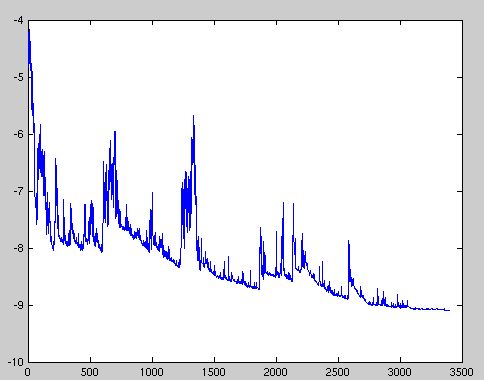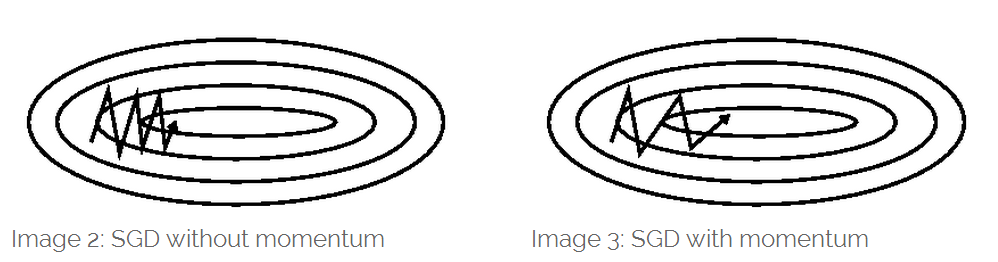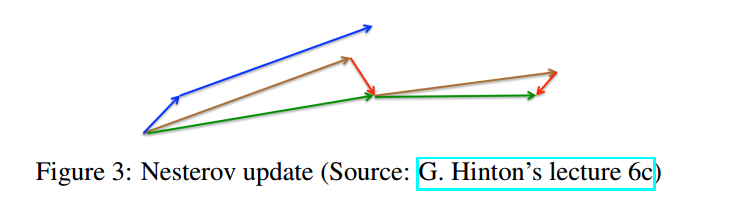# 梯度下降算法

## 批量梯度下降

Vanilla梯度下降，也叫作批量梯度下降。在整个训练数据集范围，计算损失函数的梯度，并用于更新参数 θ \theta
θ = θ − η ⋅ ▽ θ J ( θ ) \theta = \theta-\eta \cdot \triangledown _{\theta }J(\theta)

for i in range(nb_epochs):
params = params - learning_rate * params_grad


## 随机梯度下降

θ = θ − η ⋅ ▽ θ J ( θ ; x ( i ) ; y ( i ) ) \theta = \theta-\eta \cdot \triangledown _{\theta }J(\theta;x(i);y(i))for i in range(nb_epochs):
np.random.shuffle(data)
for example in data:
params = params - learning_rate * params_grad


## 小批量梯度下降

θ = θ − η ⋅ ▽ θ J ( θ ; x ( i : i + n ) ; y ( i : i + n ) ) \theta = \theta-\eta \cdot \triangledown _{\theta }J(\theta;x(i:i+n);y(i:i+n))

for i in range(nb_epochs):
np.random.shuffle(data)
for batch in get_batches(data, batch_size=50):
params = params - learning_rate * params_grad


## 困难和挑战

1.选择一个合适的学习率比较困难，学习率过小会导致收敛很慢，学习率过大会妨碍收敛，导致损失函数在最小值点出波动设置导致发散。
2.学习率表（就好比一个根据时间或步数规划好的表）尝试在训练期间调整学习率，比如退火算法。即根据预先设定好的计划表逐步降低学习率；或者当目标函数低于某些设定好的阈值的时候改变学习率。然而这种根据步数或者阈值的方法都需要提前定义好，因为无法自适应不同数据集的特征。
3.此外，相同的学习率应用到所有的参数。但是如果我们的数据是稀疏的，特征有非常不同的频率，这样的话我们并不想把每一个特征都更新到相同的程度。
4.最后一个很关键的困难在于在神经网络中最小化非凸误差函数面要避免陷入诸多的局部最小值中，而Dauphin等人认为困难实际上不是来自于局部最小值的问题，而是来自于鞍点，鞍点即一个维度向上倾斜并且在另一个维度向下倾斜的点。 这些鞍点通常被相同误差的平面所围绕，这使得SGD很容易陷入鞍点，因为在鞍点处的每一个维度的梯度都接近于零。

# 梯度下降优化算法

## 动量v t = γ v t − 1 + η ⋅ ▽ θ J ( θ ) v_{t} = \gamma v_{t-1}+\eta \cdot \triangledown _{\theta }J(\theta)

θ = θ − v t \theta = \theta-v_{t}

## Nesterov 加速梯度

Nesterov 加速梯度（NAG）算法提供了一种对动量预测的方法。我们知道依靠动量 γ v t − 1 \gamma v_{t-1} 来改变参数 θ \theta 。计算 θ − γ v t − 1 \theta- \gamma v_{t-1} 给出了参数的下一个位置的近似值（完全更新缺失的梯度），这种估计方法比较粗略。然而，我们不计算关于当前参数的梯度而是计算关于参数的大致未来位置的梯度，这样的话能够有效的预测。

v t = γ v t − 1 + η ⋅ ▽ θ J ( θ − γ v t − 1 ) v_{t} = \gamma v_{t-1}+\eta \cdot \triangledown _{\theta }J(\theta - \gamma v_{t-1})

θ = θ − v t \theta = \theta-v_{t}g t , i = ▽ θ t J ( θ t , i ) g_{t,i} = \triangledown _{\theta_{t} } J(\theta _{t},i)

θ t + 1 , i = θ t , i − η ⋅ g t , i \theta _{t+1},i = \theta _{t},i - \eta \cdot g_{t,i}

θ t + 1 , i = θ t , i − η G t , i i + ϵ ⋅ g t , i \theta _{t+1},i = \theta _{t},i - \frac{\eta}{\sqrt{G_{t,ii}+\epsilon }} \cdot g_{t,i}

10-19511
04-143万+11-12112
10-038932
07-211万+
03-249955
11-299万+
09-18885
11-262万+
©️2020 CSDN 皮肤主题: Age of Ai 设计师:meimeiellie